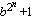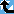# Generalized Fermat Divisors (base=12)

The Prime Pages keeps a list of the 5000 largest known primes, plus a few each of certain selected archivable forms and classes. These forms are defined in this collection's home page.

The numbers Fb,n =(with b an integer greater than one) are called the generalized Fermat numbers. (In the Prime database they are denoted GF(n,b) to avoid the use of subscripts.) It is reasonable to conjecture that for each base b, there are only finitely many such primes.

As in the case of the Fermat numbers, many have interested in the form and distribution of the divisors of these numbers. When b is even, each of their divisors must have the form

k.2m+1
with k odd and m>n. For this reason, when we find a large prime of the form k.2n+1 (with k small), we usually check to see if it divides a Fermat number. For example, Gallot's Win95 program Proth.exe has this test built in for a few choices of b.

The number k.2n+1 (k odd) will divide some generalized Fermat number for roughly 1/k of the bases b.

###Record Primes of this Type

rankprime digitswhowhencomment
17 · 220267500 + 1 6101127 L4965 Jul 2022 Divides GF(20267499, 12) [GG]
29 · 211500843 + 1 3462100 L4965 Mar 2020 Divides GF(11500840, 12)
325 · 28456828 + 1 2545761 L5237 Jan 2021 Divides GF(8456827, 12), generalized Fermat
411 · 28103463 + 1 2439387 L4965 Mar 2020 Divides GF(8103462, 12)
539 · 27946769 + 1 2392218 L5226 Jan 2021 Divides GF(7946767, 12)
651 · 26945567 + 1 2090826 L4965 May 2020 Divides GF(6945564, 12) [p286]
781 · 25115131 + 1 1539810 L4965 Aug 2022 Divides GF(5115128, 12) [GG]
8123 · 23230548 + 1 972494 L5178 Apr 2022 Divides GF(3230546, 12)
97 · 22915954 + 1 877791 g279 Jun 2008 Divides GF(2915953, 12) [g322]
1011 · 22691961 + 1 810363 p286 Mar 2013 Divides GF(2691960, 12)
119 · 22543551 + 1 765687 L1204 Jun 2011 Divides Fermat F(2543548), GF(2543549, 3), GF(2543549, 6), GF(2543549, 12)
123 · 22478785 + 1 746190 g245 Oct 2003 Divides Fermat F(2478782), GF(2478782, 3), GF(2478776, 6), GF(2478782, 12)
133 · 22145353 + 1 645817 g245 Feb 2003 Divides Fermat F(2145351), GF(2145351, 3), GF(2145352, 5), GF(2145348, 6), GF(2145352, 10), GF(2145351, 12)
147 · 22139912 + 1 644179 g279 May 2007 Divides GF(2139911, 12)
15101 · 21988279 + 1 598534 L3141 May 2013 Divides GF(1988278, 12)
16129 · 21774709 + 1 534243 L2526 Mar 2013 Divides GF(1774705, 12)
1763 · 21686050 + 1 507554 L2085 Aug 2011 Divides GF(1686047, 12)
1855 · 21669798 + 1 502662 L2518 Aug 2011 Divides GF(1669797, 12)
195 · 21320487 + 1 397507 g55 Mar 2002 Divides GF(1320486, 12)
2025 · 21211488 + 1 364696 g279 Jan 2005 Generalized Fermat, divides GF(1211487, 12)

###References

BR98
A. Björn and H. Riesel, "Factors of generalized Fermat numbers," Math. Comp., 67 (1998) 441--446.  MR 98e:11008 (Abstract available)
DK95
H. Dubner and W. Keller, "Factors of generalized Fermat numbers," Math. Comp., 64 (1995) 397--405.  MR 95c:11010
RB94
H. Riesel and A. Börn, Generalized Fermat numbers.  In "Mathematics of Computation 1943-1993: A Half-Century of Computational Mathematics," W. Gautschi editor, Proc. Symp. Appl. Math. Vol, 48, Amer. Math. Soc., Providence, RI, 1994.  pp. 583-587, MR 95j:11006
Riesel69b
H. Riesel, "Common prime factors of the numbers An =a2n+1," BIT, 9 (1969) 264-269.  MR 41:3381